12-34.

Missing problem for Book CCA2 => Chapter Ch12 => Lesson 12.1.2 => Problem 12-34

Created from orphaned homework help problem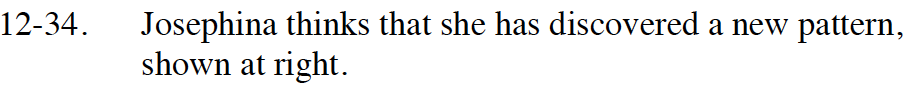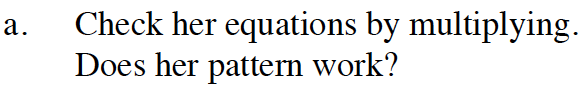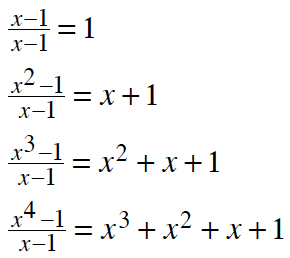To check a division problem using multiplication, multiply the divisor by the quotient. In the second case, multiply (x − 1)(x + 1). Does it equal x2 − 1? How will you check the third and fourth cases?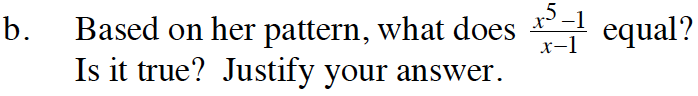Look for a pattern in the quotients. Look at the number of terms and the exponent each term.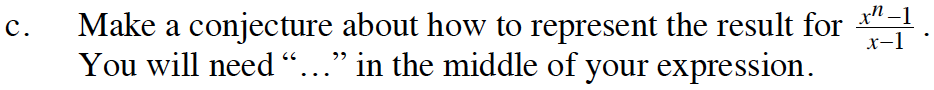$x^n + x^{n-1} + x^{n-2} +...+ x + 1$